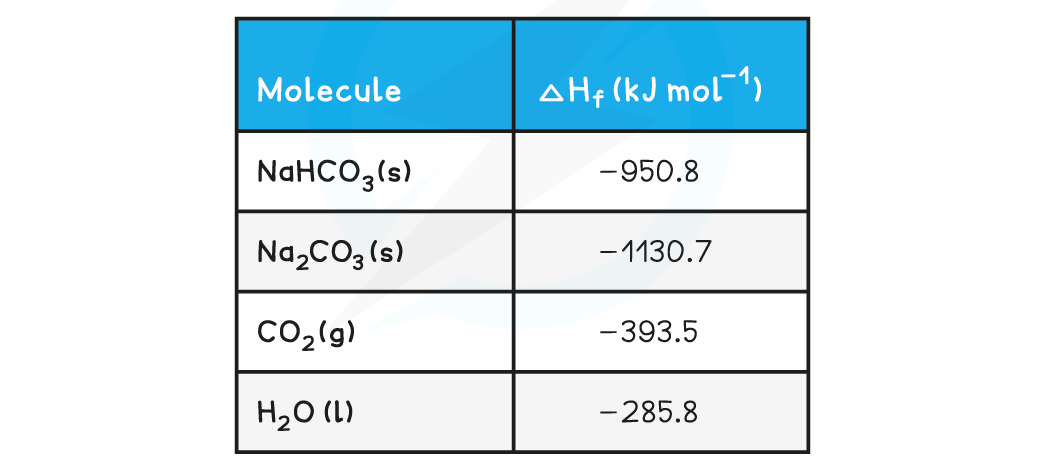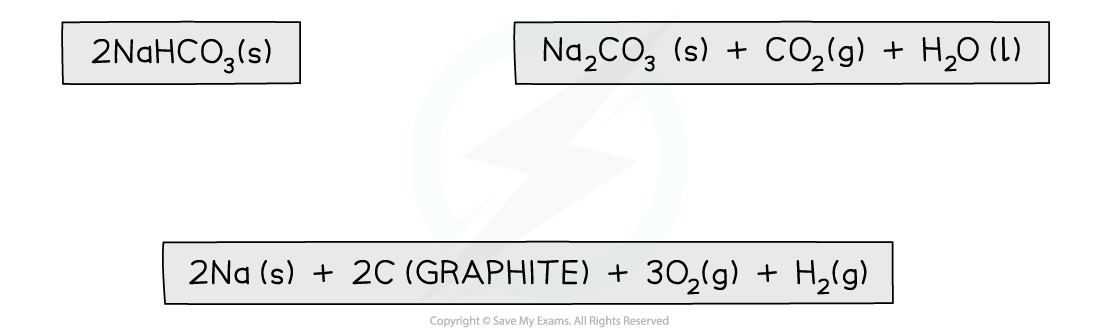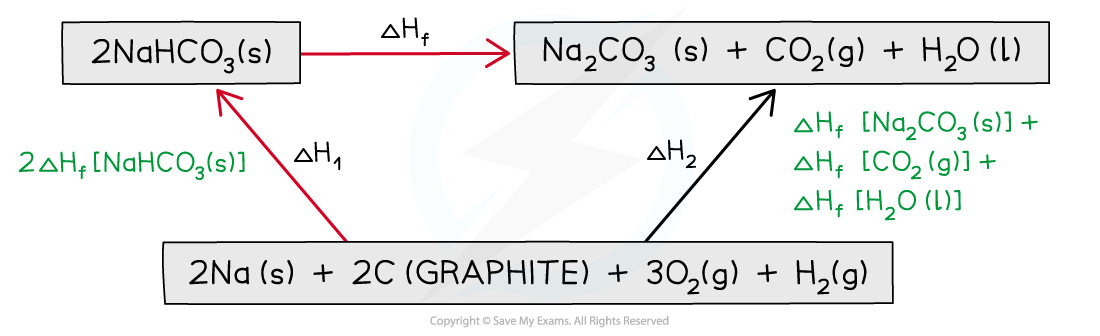# Edexcel A Level Chemistry:复习笔记1.8.4 Hess Cycles

### Constructing Hess Cycles

• In 1840, the Russian chemist Germain Hess formulated a law which went on to be known as Hess’s Law
• This went on to form the basis of one of the laws of thermodynamics. The first law of thermodynamics relates to the Law of Conservation of Energy
• It is sometimes expressed in the following form:

Energy cannot be created or destroyed, it can only change form

• This means that in a closed system, the total amount of energy present is always constant
• Hess’s law can be used to calculate the standard enthalpy change of a reaction from known standard enthalpy changes
• Hess’s Law states that:

"The total enthalpy change in a chemical reaction is independent of the route by which the chemical reaction takes place as long as the initial and final conditions are the same."

• This means that whether the reaction takes place in one or two steps, the total enthalpy change of the reaction will still be the sameThe diagram above illustrates Hess’ Law: the enthalpy change of the direct route, going from reactants (A+B) to product (C) is equal to the enthalpy change of the indirect routes

• Hess’ Law is used to calculate enthalpy changes which can’t be found experimentally using calorimetry, e.g.:

3C (s) + 4H2 (g) → C3H8(g)

• ΔfH (propane) can’t be found experimentally as hydrogen and carbon don’t react under standard conditions

#### Calculating ΔrH from ΔfH using Hess’s Law energy cycles

• You can see the relationships on the following diagram:The enthalpy change from elements to products (direct route) is equal to the enthalpy change of elements forming reactants and then products (indirect route)

• The products can be directly formed from the elements = ΔH2

OR

• The products can be indirectly formed from the elements = ΔH1 + ΔHr
• Equation

ΔH2 = ΔH1 + ΔHr

Therefore for energy to be conserved,

ΔHr = ΔH2 – ΔH1

#### Exam Tip

You do not need to learn Hess's Law word for word as it is not a syllabus requirement, but you do need to understand the principle as it provides the foundation for all the problem solving in Chemical Energetics

### Hess Cycle Calculations

• Hess cycles can be used to calculate various enthalpy changes as long as sufficient information about the other sides of the cycle is known

#### Worked Example

Calculating the enthalpy change of reaction

Calculate the ΔHf for the following reaction:

2NaHCO3 (s)  → Na2CO3 (s) + CO2 (g) + O(I)

The table below shows the standard enthalpy of formations (ΔHfꝋ) relevant to this reaction:Step 1: Write the balanced equation at the topStep 2: Draw the cycle with the elements at the bottomStep 3: Draw in all arrows, making sure they go in the correct directions. Write the standard enthalpy of formationsStep 4: Apply Hess’s Law

• ΔrH = ΔH2 - ΔH1
• It is minus ΔH1 because you have to go in the opposite direction of the arrow
• ΔH2 is ΔfH [Na2CO3 (s)] + ΔfH [CO2 (g)] + ΔfH [H2O (l)]
• ΔH1 is 2 x ΔfH [NaHCO3 (s)]
• ΔrH = (ΔfH [Na2CO3 (s)] + ΔfH [CO2 (g)] + ΔfH [H2O (l)]) - (2ΔfH [NaHCO3 (s)])
• ΔrH = ((-1130.7) + (-393.5) + (-285.8)) - (2 x (-950.8))
• ΔrH = +91.6 kJ mol-1

#### Exam Tip

Keep your enthalpy values inside their own brackets so that you don't accidentally lose a minus sign